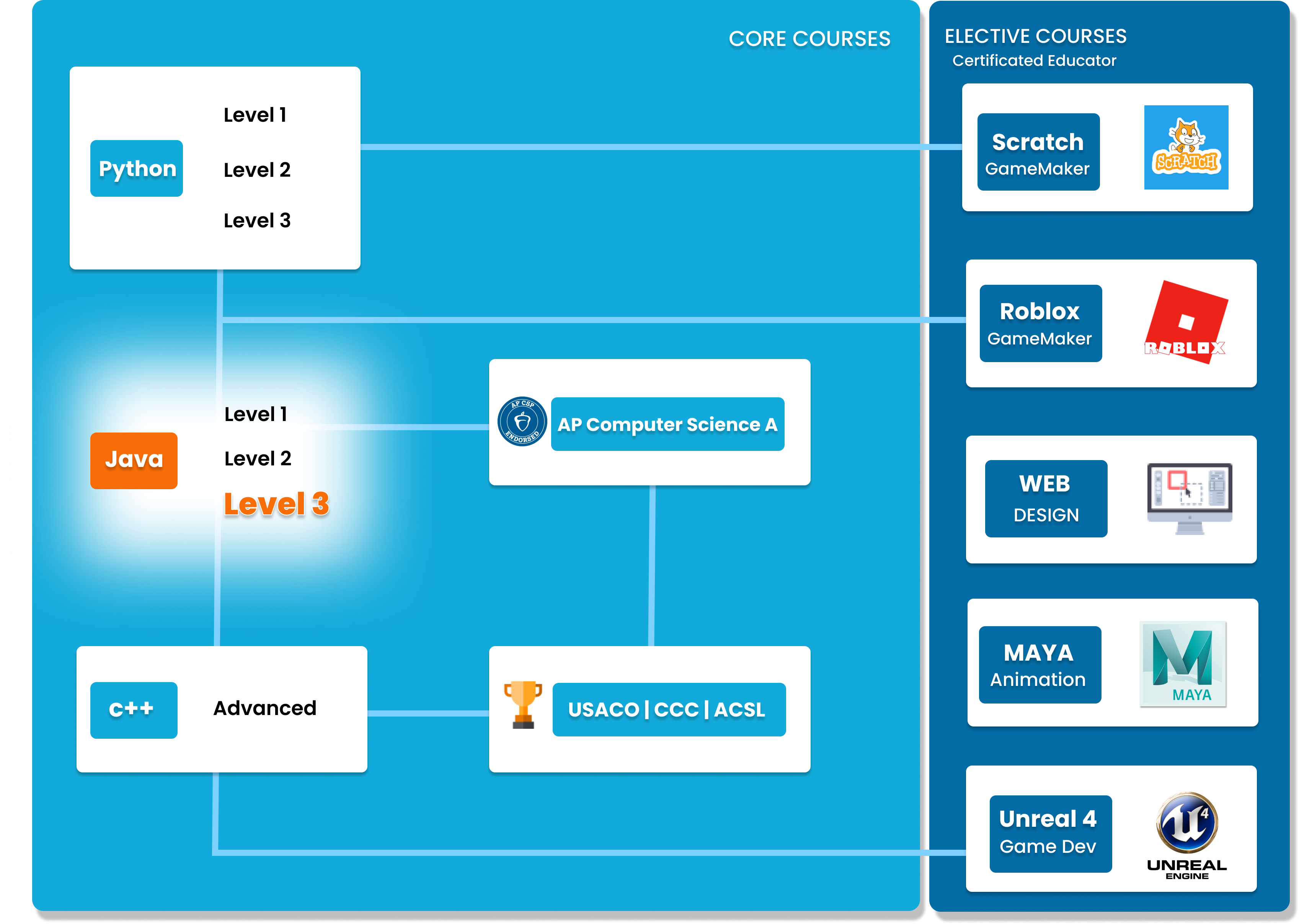# Java Level 3

In this advanced Java course, students will build on the concepts covered in level 2. It is expected that students have either taken Java level 2, or have a strong understanding of the concepts covered in this level. Concepts covered in this course include program design and analysis, recursion, sorting and searching and more. Students will apply these concepts to creating advanced games, such as Chess. By the end of this course students will have an advanced understanding of Java. Strong students can choose to take our AP Java course next - an accelerated curriculum that teaches Java to a university level understanding.##### Who is this course for
Intermediate Students: This course is for students who have completed both Level 1 and 2 or have previous experience in coding.
This course typically takes 3-6 months to complete. This depends on the student's experience and how fast they can master the concepts and the knowledge. Our instructors move at the pace of the student, it may take extra time for some students to reinforce what they have learned.
##### Curriculum
###### Chapter 1: Arrays and ArrayListsChapter 1 introduces the Array and ArrayList data structures in Java. Students will learn how to create, modify, and manipulate Arrays and ArraysLists. Additionally, students will learn about the List interface, Collections, Iterators, and dive into two-dimensional arrays.
###### Chapter 2: RecursionChapter 2 features the alternative type of loop: recursion. Students will learn how to use recursion and how it differs from iteration.
###### Chapter 3: Sorting and SearchingChapter 3 focuses on the basics of Sorting and Searching. Students will learn about Selection Sort, Insertion Sort, Merge Sort, Quicksort, Sequential Search, and Binary Search.
###### Chapter4: Introduction to threads and concurrencyYou will learn the basics of MultiThreaded Programming - with Executor Service
###### Chapter 5: Capstone ProjectChoose 1 of 3 capstone projects. Use all knowledge to complete your favourite project.

### You Might Also Be Interested In Our Elective Courses##### Introduction to Number Theory
AGES 10 - 15

This course is designed to build the basics of a complicated mathematical field. It is designed to be a complete introduction to the fundamental concepts of number theory for students in grades 7-10.

The class covers topics such as primes and composites; multiples, divisors, and divisibility; prime factorization and its uses; modular arithmetic and more.##### Introduction to Geometry
AGES 10 - 15

Students in grades 6-10 will build a solid understanding of more advanced geometry concepts in this course. It is designed to expand on the standard high school geometry curriculum and to challenge students with new concepts and problems.

Topics include congruent and similar triangles, circles, 3D geometry, and introductory trigonometry.##### Introduction to Counting and Probability
AGES 10 - 15

To build on the concepts from the Introduction level course, students will build on their Counting and Probability knowledge.

A thorough introduction for students in grades 7-10 to counting and probability topics such as permutations, combinations, Pascal's triangle, geometric probability, basic combinatorial identities, the Binomial Theorem, Pigeonhole Principle Conditional, Probability Graph Theory and more.